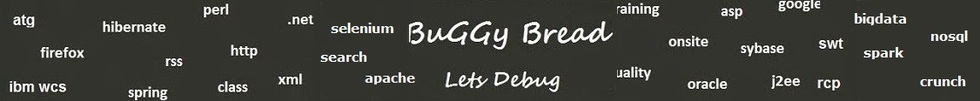## Search Java Classes and Packages

Search Java Frameworks and Libraries

255581 classes and counting ...
 Search Tips Index Status

#Org.uacalc.util Classes and Interfaces - 7 results found.
 Name Description Type Package Framework ArrayIncrementor This is for in place incrementing of an array. Interface org.uacalc.util UACalc ( Universal Algebra Calculator ) ArrayString A static method to print out an array based on Java Tech Tips, Feb 5, 2002. Class org.uacalc.util UACalc ( Universal Algebra Calculator ) Horner This has static methods for the Horner encoding and its inverse. Class org.uacalc.util UACalc ( Universal Algebra Calculator ) IntArray This class is a wrapper for an array of int's mainly so we can specify the equals and hashCode methods. Class org.uacalc.util UACalc ( Universal Algebra Calculator ) PermutationGenerator This class is used to help generate permutations of arrays. Class org.uacalc.util UACalc ( Universal Algebra Calculator ) SequenceGenerator This has utility static methods for sequence generation, including nondecreasing sequences. Class org.uacalc.util UACalc ( Universal Algebra Calculator ) SimpleList Simple Linked lists. Class org.uacalc.util UACalc ( Universal Algebra Calculator )